# Logic Simplifications A+ A  A-

In Boolean algebra, a sum term is a sum of literals. In logic circuits, a sum term is produced by an OR operation with no AND operations involved.

Boolean Multiplication
Boolean multiplication is equivalent to the AND operation. In Boolean algebra, a product term is the product of literals. In logic circuits, a product term is produced by an AND operation with no OR operations involved

LAWS AND RULES OF BOOLEAN ALGEBRA

Laws of Boolean Algebra
The basic laws of Boolean algebra-the commutative laws for addition and multiplication, the associative laws for addition and multiplication, and the distributive law-are the same as in ordinary algebra.

Commutative Laws

• The commutative law of addition for two variables is written as A+B = B+A
This law states that the order in which the variables are ORed makes no difference.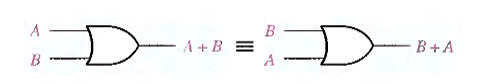• The commutative law of multiplication for two variables is  A.B = B.A
This law states that the order in which the variables are ANDed makes no differenceAssociative Laws :

• The associative law of addition is written as follows for three variables:  A + (B + C) = (A + B) + C
This law states that when ORing more than two variables, the result is the same regardless of the grouping of the         variables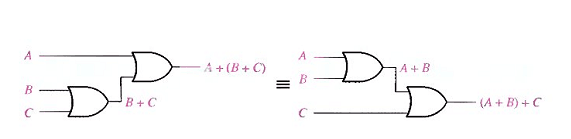• The associative law of multiplication is written as follows for three variables: A(BC) = (AB)C

This law states that it makes no difference in what order the variables are grouped when ANDing more than two     variables.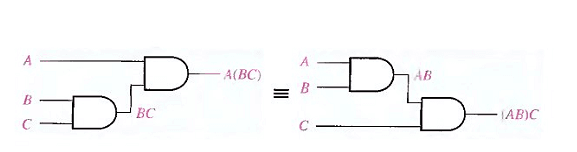Distributive Law:

• The distributive law is written for three variables as follows:
A(B + C) = AB + AC
This law states that ORing two or more variables and then ANDing the result with a single variable is equivalent to     ANDing the single variable with each of the two or more variables and then ORing the products. The distributive law also expresses the process of factoring in which the common variable A is factored out of the product terms,    for example,
AB + AC = A(B + C).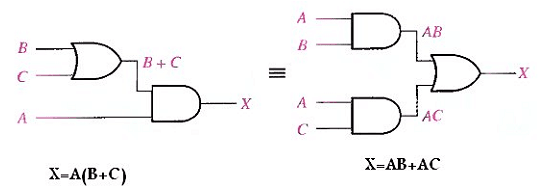Rules of Boolean Algebra

lists 12 basic rules that are useful in manipulating and simplifying Boolean expressions. Rules 1 through 9 will be viewed in terms of their application to logic gates. Rules 10 through 12 will be derived in terms of the simpler rules and the laws previously discussed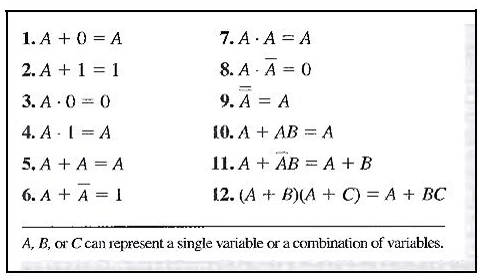DEMORGAN’S THEOREMS

DeMorgan, a mathematician who knew Boole, proposed two theorems that are an important part of Boolean algebra. In practical terms. DeMorgan’s theorems provide mathematical verification of the equivalency of the NAND and negative-OR gates and the equivalency of the NOR and negative-AND gates

One of DeMorgan’s theorems is stated as follows:

The complement of a product of variables is equal to the sum of the complements of the variables,
Stated another way, The complement of two or more ANDed variables is equivalent to the OR of the complements of the individual variables.
The formula for expressing this theorem for two variables is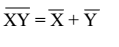DeMorgan’s second theorem is stated as follows:

The complement of a sum of variables is equal to the product of the complements of the variables.
Stated another way, The complement of two or more ORed variables is equivalent to the AND of the complements of the individual variables,
The formula for expressing this theorem for two variables is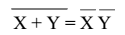shows the gate equivalencies and truth tables for the two equations above.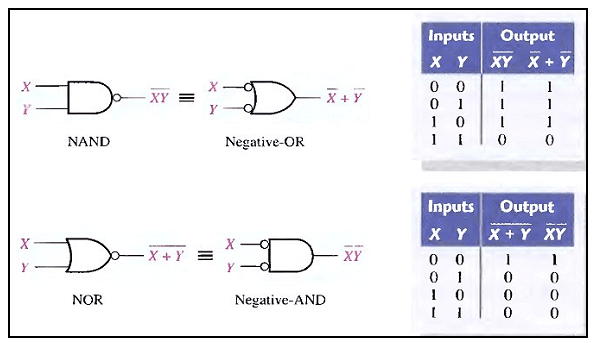SIMPLIFICATION USING BOOLEAN ALGEBRA

A simplified Boolean expression uses the fewest gates possible to implement a given expression.
Example

Using Boolean algebra techniques, simplify this expression:
AB + A(B + C) + B(B + C)

Solution

Step 1: Apply the distributive law to the second and third terms in the expression, as follows:
AB + AB + AC + BB + BC

Step 2: Apply rule 7 (BB = B) to the fourth term.
AB + AB + AC + B + BC

Step 3: Apply rule 5 (AB + AB = AB) to the first two terms.
AB + AC + B + BC

Step 4: Apply rule 10 (B + BC = B) to the last two terms.
AB + AC + B

Step 5: Apply rule 10 (AB + B = B) to the first and third terms.
B+AC

At this point the expression is simplified as much as possible.STANDARD FORMS OF BOOLEAN EXPRESSIONS

All Boolean expressions, regardless of their form, can be converted into either of two standard forms:

the sum-of-products form or the product-of sums form. Standardization makes the evaluation, simplification, and implementation of Boolean expressions much more systematic and easier.

The Sum-of-Products (SOP) Form
When two or more product terms are summed by Boolean addition, the resulting expression is a sum-of-products (SOP). Some examples are: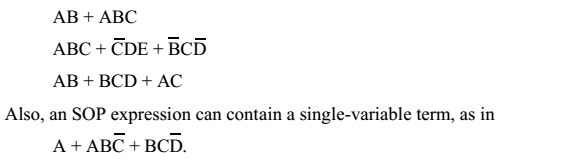In an SOP expression a single overbar cannot extend over more than one variable.

The Product-of-Sums (POS) Form
A sum term was defined before as a term consisting of the sum (Boolean addition) of literals (variables or their complements).

When two or more sum terms are multiplied, the resulting expression is a product-of-sums (POS).

Some examples are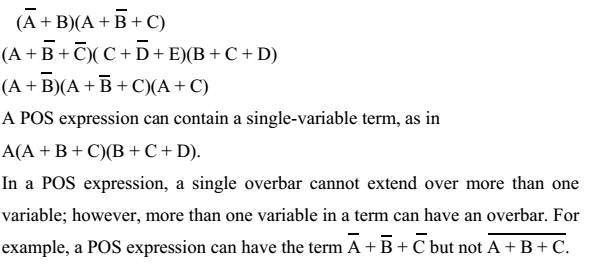Implementation of a POS Expression simply requires ANDing the outputs of two or more OR gates. A sum term is produced by an OR operation and the product of two or more sum terms is produced by an AND operation

KARNAUGH MAP MINIMIZATION

A Karnaugh map provides a systematic method for simplifying Boolean expressions and, if properly used, will produce the simplest SOP or POS expression possible, known as the minimum expression. As you have seen,
the effectiveness of algebraic simplification depends on your familiarity with all the laws, rules, and theorems of Boolean algebra and on your ability to apply them. The Karnaugh map, on the other hand, provides a “cookbook”
method for simplification.

A Karnaugh map is similar to a truth table because it presents all of the possible values of input variables and the resulting output for each value. Instead of being organized into columns and rows like a truth table, the Karnaugh map is an array of cells in which each cell represents a binary value of the input variables.

The cells are arranged in a way so that simplification of a given expression is simply a matter of properly  grouping the cells. Karnaugh maps can be used for expressions with two, three, four. and five variables. Another method, called the Quine-McClusky method can be used for higher numbers of variables.

The number of cells in a Karnaugh map is equal to the total number of possible input variable combinations as is the number of rows in a truth table. For three variables, the number of cells is 2^3 = 8. For four variables, the number of cells is 2^4 = 16.